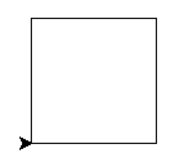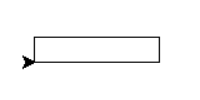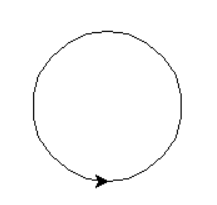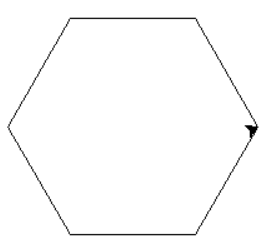# How to draw different shapes using the Python Turtle library?

In this program, we will draw different shapes using the Turtle library in Python. Turtle is a python feature like a drawing board, which lets you command a turtle to draw all over it. The different shapes that we are going to draw are square, rectangle, circle and a hexagon.

## Algorithm

Step 1: Take lengths of side for different shapes as input.

Step 2: Use different turtle methods like forward() and left() for drawing different shapes.

## Example Code

import turtle
t = turtle.Turtle()

#SQUARE
side = int(input("Length of side: "))
for i in range(4):
t.forward(side)
t.left(90)

#RECTANGLE
side_a = int(input("Length of side a: "))
side_b = int(input("Length of side b: "))
t.forward(side_a)
t.left(90)
t.forward(side_b)
t.left(90)
t.forward(side_a)
t.left(90)
t.forward(side_b)
t.left(90)

#CIRCLE

#HEXAGON
for i in range(6):
t.forward(side)
t.left(300)

## Output

SQUARE:
Length of side: 100RECTANGLE:
Length of side a: 100
Length of side b: 20CIRCLE:HEXAGON:Length of side: 100